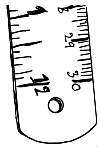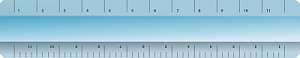# Centimeters to Inches Calculator

Instructions: Use this calculator to convert a centimeters to feet, showing all the steps. Please type the number of centimeters you would like to convert in the form below:Type the number of centimeters:

## Using this Centimeter to Inches Conversion Calculator

It is not unusual to be in need to making unit conversions. When our friend from overseas tells us they are 1.83 meters tall, maybe we will ask him, "what is that in feet and inches?"

Perhaps we have an idea of approximately how tall 1.83 meters is, but we are not clear about precisely how much. So we will want to have a conversion tool that can tell us much more precise amounts.## How many centimeters are there in an inch?

There is a basic equivalence between centimeters and inches that can be reflected down to the basic units. Indeed, we have that 1 inch is approximately the same as 2.54 centimeters. Using this basic equivalence between fundamental units will be crucial when deriving a general conversion formula.

## How do you convert centimeters to inches?

The conversion of units is quite simple, and it should not present many complications. Now, sometimes it will be hard to straight up memorize the formula. It is usually a better idea to understand the rationale used to derive the formulas.

### Steps for the conversion of centimeters to Feet

1. Notice that 1 inch is the same as 2.54 centimeters. This is used as the initial proportion
2. Assume you have given C centimeters. You want to know many inches $$I$$ are equivalent to those given C centimeters
3. Using direct proportion, we establish that $$\displaystyle \frac{1}{2.54} = \frac{I}{C}$$
4. Solving the proportion for I, we get the following conversion formula for inches: $$I = \displaystyle \frac{C}{2.54}$$

With this formula, all you need to do is to plug the known number of centimeters into the formula, and you are good to go.### Examples: Converting feet and inches to centimeters

What is 176 cms in inches?

Solution: We need to use the conversion formula:

$F = \displaystyle \frac{C}{2.54}$

In this case, the given length in centimeters is C = 176 cms, so then plugging it in the above formula we find that :

$F = \displaystyle \frac{C}{2.454} = \displaystyle \frac{176}{2.54} = 69.291 \text{ inches}$

### Another conversion example

Convert 150 cms in inches

Solution: We use the same procedure. We use the conversion formula:

$F = \displaystyle \frac{C}{2.54}$

and now the given length is C = 150 cms and by plugging it in the formula, we find that:

$F = \displaystyle \frac{C}{2.54} = \displaystyle \frac{150}{2.54} = 59.055 \text{ feet}$

## Conversion calculators

There are many other metric conversion tools that you can find on our site. You can check out our meters to inches calculator, or the centimeters to feet calculator, or also our meters to feet, imperial to centimeters or imperial to meters calculators, based on the type of calculation/conversion you need to conduct.